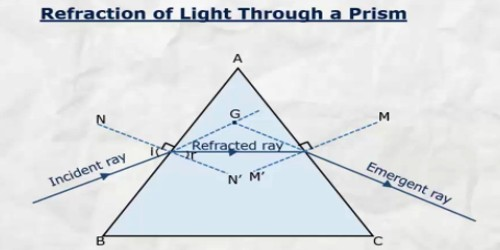Physics

# Refraction of Light through PrismRefraction of Light through Prism

A transparent homogeneous medium bounded by three mutually intersecting plane surfaces is called a prism. The surface through which light enters the prism and the surface through which light emerges are called refracting surfaces of the prism. Suppose ABC is the principal section of a prism. AB and AC are refracting surfaces, ∠A, the angle of prism and ABC is the base of the prism [Figure].Let an incident ray PQ be incident obliquely from the air at point Q on the surface of the prism AB. In this case, since the ray is incident from rarer medium to denser medium, the refracted ray moves away from the normal NQOʹ drawn at point Q of the surface AB and refracted along the path QR. Afterward, this ray will be incident at point R of the surface AC and again emerges into air medium along the path RS. Then again the ray will be refracted and while going from glass to air medium the refracted ray will move away from the normal NʹR drawn at point R on the surface AC. Here PQRS represents the path of the ray of light. If there would have been no prism, the incident ray PQ would have gone straight. Due to the presence of the prism the path of the ray of light has been changed i.e., the ray of light has deviated. Now, if the incident ray PQ is extended forward up to ‘L’ and the emerging ray RS is extended backward, then these two rays will meet at point O. Here angle ∠SOL represents the angle of deviation for that ray. It is denoted by δ or D.

∠SOL = δ or D.

Definition of the angle of deviation: If the incident ray on the prism is extended forward and the emerging ray from the prism is extended backward, then the angle between these two rays is called the angle of deviation or deviation. In short, the angle between the ray of incidence and the ray of emergence is called the angle of deviation. It is denoted by δ or D.

Drawing: Let the ray NʹR due to extension backward meet with NQOʹ at point O.

Calculation of deviation: Suppose ∠PQN = i1, ∠OʹQR = r1, ∠SRNʹ = i2, and ∠OʹRQ = r2.

Then total deviation, δ = Q deviation at point Q + deviation at point R.

or, δ = x + xʹ = (i1 – r1) + (i2 – r2)

or, δ = (i1 + i2) (r1 + r2) …. …. …. (1)

Now, in triangle OʹQR, ∠Oʹ + ∠r1 + ∠r2 = two right angles … … … (2)

Again, in quadrilateral AQOʹR, ∠AQOʹ = ∠AROʹ = one right angle.

So, ∠A + ∠Oʹ = two right angles … … … (3)

From equations (2) and (3) we get,

∠A + ∠Oʹ = ∠Oʹ + ∠r1 + ∠r2

So, ∠A = ∠r1 + ∠r2

Now inserting the values of (r1 + r2) in equation (1) we get, angle of deviation or deviation,

δ = i1 + i2A

This is the equation of the angle of deviation of light through a prism.

So, by knowing the first angle of incidence, the second angle of retraction and the angle of prism we can determine the angle of deviation of light passing through a prism.